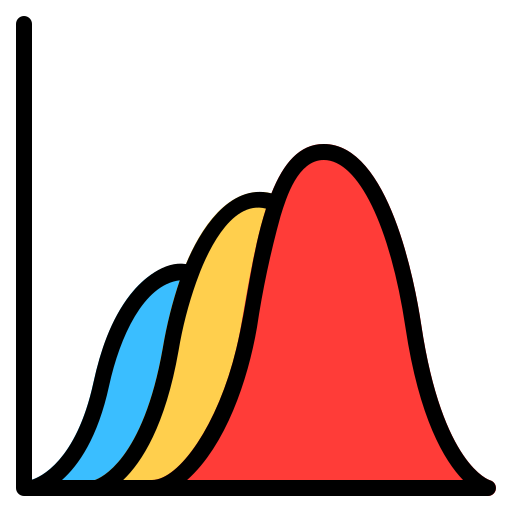# ProbabilityJay

Published May 17, 2021

6 Courses

Statistics
Probability

## Overview and objectives

In this course we'll focus on probability theory and move towards how to tackle different types of probability questions.

## Audience

The pre-requisites for this course involve basic understanding of probability and statistics. Some concepts may be difficult without a college level understanding of math.

## Courses

Courses in this learning path are:### Basic Probability

Probability Theory is the branch of mathematics that deals with uncertainty, underpinning all of statistics and machine learning.

0 of 10 Completed### Discrete Distributions

All areas of study in math can roughly be divided into two camps: discrete mathematics and continuous mathematics. Perhaps the best way to describe the difference between the two is to talk about what each of the branches means by "number."

1 of 12 Completed### Continuous Distributions

Continuous probability distribution: A probability distribution in which the random variable X can take on any value (is continuous).

1 of 6 Completed### Multivariate Distributions

Multivariate distributions show comparisons between two or more measurements and the relationships among them. For each univariate distribution with one random variable, there is a more general multivariate distribution.

1 of 9 Completed### Sampling Theorems

Thus far in this course, we have considered random variables under an idealized scenario where we know the distribution of the random variable.

2 of 7 Completed### Probability Questions: Hard

Let's tackle harder probability questions you'd expect to see from quantitative finance or trading interviews.

3 of 5 Completed

Good job, keep it up!

## 16%

Completed

You have 41 sections remaining on this learning path.

There's so much more to Interview Query! Sign up to access hundreds of interview questions, expert coaching and a flourishing data science community.